Function Repository Resource:

Compute the additive persistence, in any base, of an integer

Contributed by: Christopher Stover
 ResourceFunction["AdditivePersistence"][n,b] gives the additive persistence of the non-negative integer n when expressed in the base b. ResourceFunction["AdditivePersistence"][n] gives the base-10 additive persistence of n.

## Details

Starting with a non-negative integer n, add its base-b digits, then add the digits of the resulting number, etc., until the result has only one digit. The number of additions required to reach the single digit stopping point is called the additive persistence of n.
As an example in base-10, consider n=1191:
Adding the digits of the result yields 1+2=3. Because 3 is a single-digit number, the process stops.
Because it took two steps to reach the stopping point, ResourceFunction["AdditivePersistence"] returns 2.

## Examples

### Basic Examples (2)

Find the additive persistence of 1191:

 In:=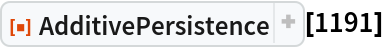Out=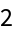Carrying out the iterated digit sum confirms this result:

 In:=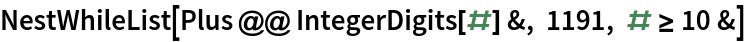Out=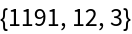Compute the additive digital root of 1899, base-14:

 In:=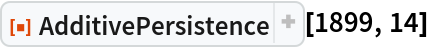Out=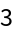### Scope (2)

 In:=Out=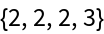In:=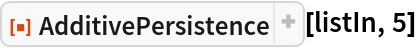Out=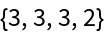Compute the additive persistence of the first 100 integers (OEIS A031286):

 In:=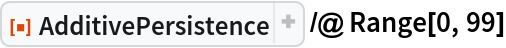Out=### Properties and Relations (3)

The result returned by AdditivePersistence can be iteratively computed using NestWhileList:

 In:=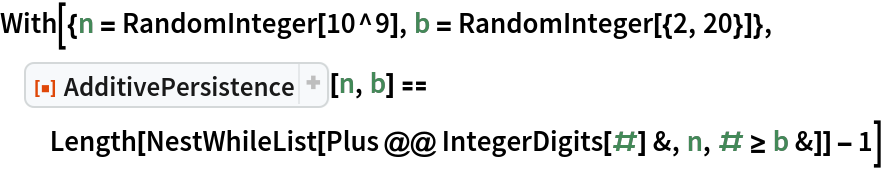Out=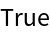The single-digit integer that marks the end of the digit addition process is called the additive digital root and is returned by the resource function AdditiveDigitalRoot:

 In:=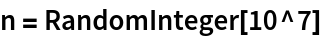Out=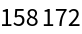In:=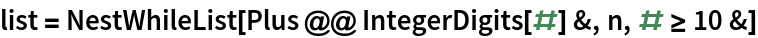Out=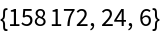In:=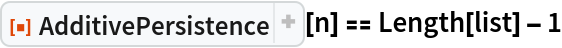Out=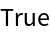In:=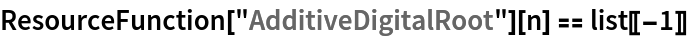Out=The multiplicative analogue of additive persistence is called multiplicative persistence and is returned by the resource function MultiplicativePersistence:

 In:=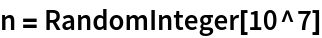Out=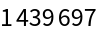In:=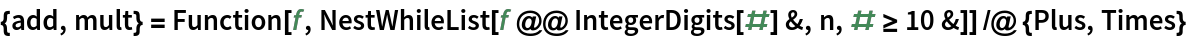Out=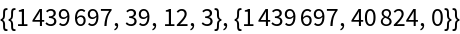In:=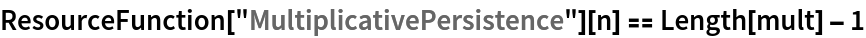Out=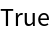### Possible Issues (1)

AdditivePersistence requires its input to be non-negative:

 In:=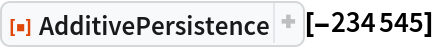Out=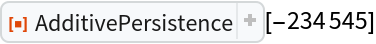### Neat Examples (1)

The smallest integers with (base-10) additive persistences equal to 0,1,2,3,4, (OEIS A006050) are:

 In:=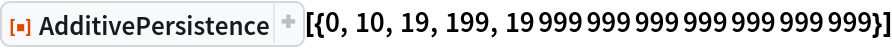Out=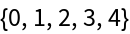The smallest integer with additive persistence 5 is known to equal 1 followed by 2222222222222222222222 9’s.

## Version History

• 1.1.0 – 25 May 2023
• 1.0.0 – 09 August 2022

## Author Notes

Future versions of this will likely contain expansion to allow for integers in bases other than 10.